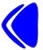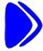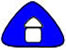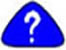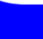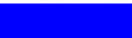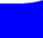Lesson 3 (page 1 of 1)

Start of Lesson 3

## Counting in Arabic

#### Objectives

This lesson covers the numbers used in the Arabic language. After completing this lesson, the user should be able to complete the following:

• Given questions about the order of Arabic numerals, the learner will be able to answer these questions correctly.
• Given questions about big numbers in Arabic numerals, the learner will be able to answer these questions correctly.
• Given questions about ordinal numbers, the learner will be able to answer these questions correctly.